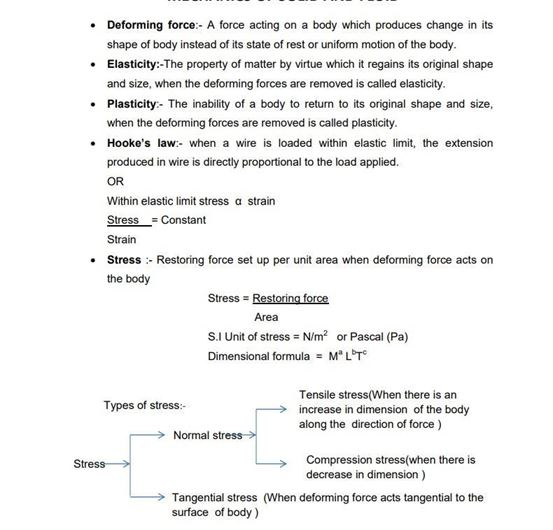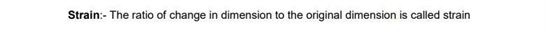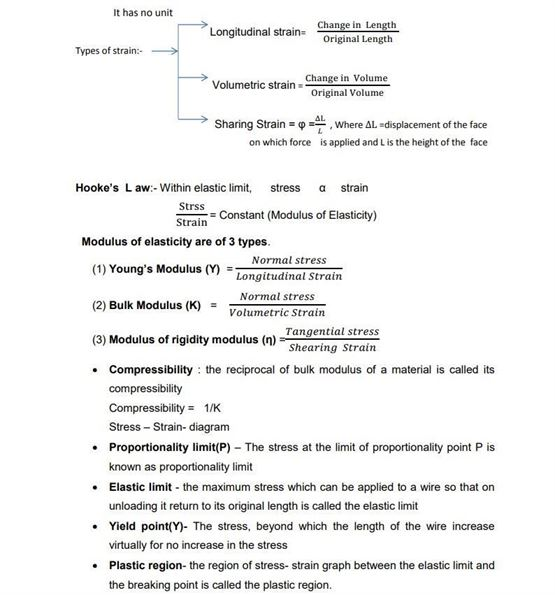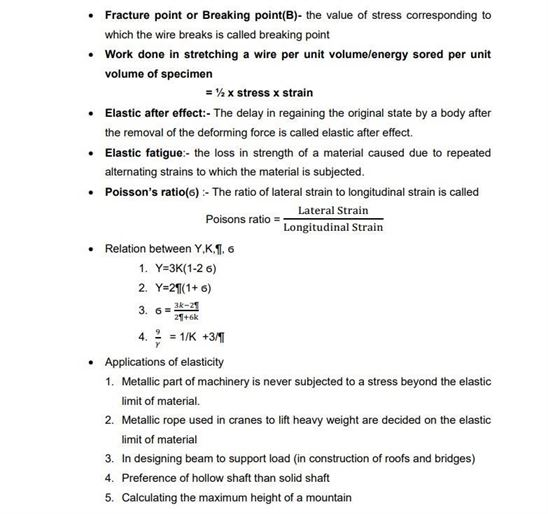# Physics Revision Notes for Class 11 Chapter 9 Mechanical Properties of Solids

Here the class 11 physics revision notes chapter 9 Mechanical Properties Of Solids is provided. These notes will be very helpful to students who can find all the important concepts related to this chapter. Students will find the definition of all the important topics in physics such as stress, plasticity, elasticity, strain etc.

• Strain is the response of a system to an applied stress. When a material is loaded with a force, it produces a stress, which then causes a material to deform. Engineering strain is defined as the amount of deformation in the direction of the applied force divided by the initial length of the material
• When a stretching force (tensile force) is applied to an object, it will extend. We can draw its force – extension graph to show how it will extend. This kind of graph is called stress- strain curve.

They will get to understand the stress- strain curve and Young’s modulus and along with that, they will also see the definition of poisson’s ratio, shear modulus and bulk modulus. These notes even contain tables showing the elastic limit, tensile strength and elastic moduli of various types of stress. Practicing from these notes will give a deep understanding in this subject,

Get the class 11 physics revision notes Chapter 9 Mechanical Properties of Solids here.